# Texas Go Math Grade 4 Lesson 9.5 Answer Key Division and the Distributive Property

Refer to our Texas Go Math Grade 4 Answer Key Pdf to score good marks in the exams. Test yourself by practicing the problems from Texas Go Math Grade 4 Lesson 9.5 Answer Key Division and the Distributive Property.

## Texas Go Math Grade 4 Lesson 9.5 Answer Key Division and the Distributive Property

Essential Question

How can you use the Distributive Property to find quotients?
The Distributive Property of division says that dividing a sum by number is the same as dividing each addend by the number and then adding the quotients.
We can use distributive property to find quotients by breaking the Dividend into two addends which can be quotients.
Explanation:
For Example;
Outline a rectangle on a grid to model 69 ÷ 3.
Shade columns of 3 until you have 69 squares.

Investigate

Materials

• color pencils
• grid paper

You can use the Distributive Property to break apart numbers to make them easier to divide.

The Distributive Property of division says that dividing a sum by a number is the same as dividing each addend by the number and then adding the quotients.

A. Outline a rectangle on a grid to model 69 ÷ 3.Shade columns of 3 until you have 69 squares.Explanation:
Outline a rectangle on a grid to model 69 ÷ 3.
Shade columns of 3 until you have 69 squares.

How many groups of 3 can you make? ____________
20 groups of 3
Explanation: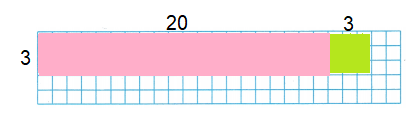B. Think of 69 as 60 + 9. Break apart the model into two rectangles to show (60 + 9) ÷ 3. Label and shade the smaller rectangles. Use two different colors.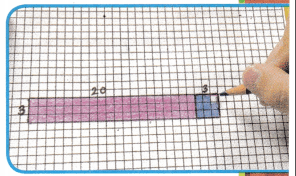Explanation:
Break apart the model into two rectangles to show (60 + 9) ÷ 3.
Label and shade the smaller rectangles.
Use two different colors to identify.

C. Each rectangle models a division.
69 ÷ 3 = (____ ÷ 3) + (________ ÷ 3)
= _________ + ___________
= ___________
69 ÷ 3 = (60 ÷ 3) + (9 ÷ 3)
= 20 + 3
= 23
Explanation:
Break apart the model into two to show
(60 + 9) ÷ 3, (9 ÷ 3)
= 20 + 3
= 23

D. Outline another model to show 68 ÷ 4.
How many groups of 4 can you make? ___________
17 groups of 4
Explanation:
Break apart the model into two to show
68 ÷ 4 = (60 ÷ 4) + (8 ÷ 4)
= 15 + 2
= 17

E. Think of 68 as 40 + 28. Break apart the model, label, and shade to show two divisions.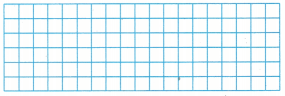68 ÷ 4 = (__________ ÷ 4) + (_________ ÷ 4)
= _________ + ___________ = __________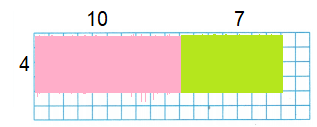68 ÷ 4 = (60 ÷ 4) + (8 ÷ 4)
= 15 + 2
= 17
Explanation:
Break apart the model into two to show
68 ÷ 4 = (60 ÷ 4) + (8 ÷ 4)
= 15 + 2
= 17

Make Connections

You can also model 68 ÷ 4 using base-ten blocks.

STEP 1 Model 68.
68 = ________ + ________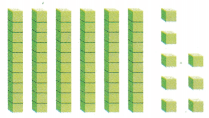68 = 40 + 28
Explanation:
Base ten is a counting system that uses ten digits to write down all numbers.
If we want to write any number, we have ten digits we can use: 0 1 2 3 4 5 6 7 8 9.
If you notice, there is no one digit for the number ten- we need to use two digits, the 1 and the 0.
We can only count to nine without the need for two digits.
Therefore, we use base ten in order to make bigger numbers.

STEP 2 Divide the longs into 4 equal groups. 4 longs divide into 4 equal groups with 2 longs left. Regroup 2 longs as 20 small cubes. Divide them evenly among the 4 groups.
60 ÷ 4 = ________Explanation:
Divide the longs into 4 equal groups.
4 longs divide into 4 equal groups with 2 longs left.
Regroup 2 longs as 20 small cubes.
Divide them evenly among the 4 groups.
We get, 15.

STEP 3 Divide the 8 small cubes into the 4 equal groups.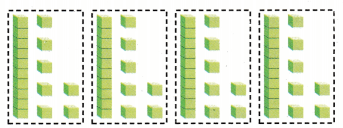8 ÷ 4 = __________

So, 68 ÷ 4 = (60 ÷ 4) + (8 ÷ 4) = _________ + _________ = _________
So, 68 ÷ 4 = (60 ÷ 4) + (8 ÷ 4) = 15 + 2 = 17
Explanation:
Divide the longs into 4 equal groups.
4 longs divide into 4 equal groups with 7 left in each group.
Divide the 8 small cubes into the 4 equal groups.

Math Talk

Mathematical Processes
Describe another way you could use the Distributive Property to solve 68 ÷ 4.

Share and Show

Model the division on the grid.

Question 1.
26 ÷ 2 = (______ ÷ 2) + (______ ÷ 2)
= ______ + ______
= ______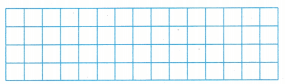26 ÷ 2 = (20 ÷ 2) + (6 ÷ 2)
= 10 + 3
= 13Explanation:
Break apart the model into two rectangles to show
26 ÷ 2 = (20 ÷ 2) + (6 ÷ 2)
= 10 + 3
= 13
Label and shade the smaller rectangles.
Use two different colors to identify.

45 ÷ 3 = (______ ÷ 3)
= ______ + ______
= ______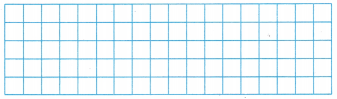45 ÷ 3 = (30 ÷ 3) + (15 ÷ 3)
= 10 + 5
= 15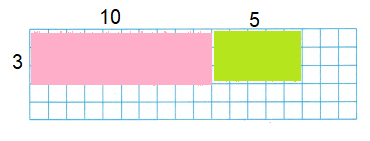Explanation:
Break apart the model into two rectangles to show
45 ÷ 3 = (30 ÷ 3) + (15 ÷ 3)
= 10 + 5
= 15
Label and shade the smaller rectangles.
Use two different colors to identify.

Question 3.
H.O.T. Evaluate To find the quotient 91 ÷ 7, would you break up the dividend into 90 .+ 1 or 70 + 21? Explain.Problem Solving.
91 ÷ 7 = (70 ÷ 7) + (21 ÷ 7)
= 10 + 3
= 13

H.O.T. Multi-Step Pose a Problem

Question 4.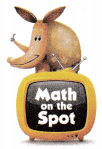Christelle went to a gift shop. The shop sells candles in a variety of sizes and colors. The picture shows a display of candles.

Write a problem that can he solved using the picture.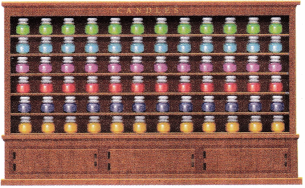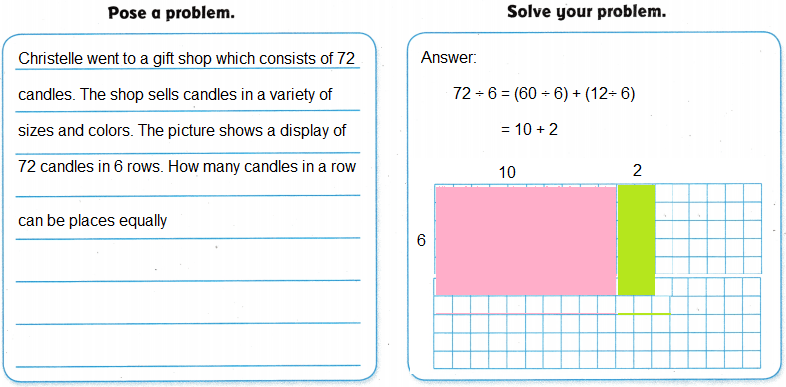Explanation:
Christelle went to a gift shop consisting of 72 candles.
The picture shows a display of 72 candles in 6 rows
Number of equal rows placed
72 ÷ 6 = 12

H.O.T. Describe how you could change the problem by changing the number of rows of candles. Then solve the problem.
72÷4 = 18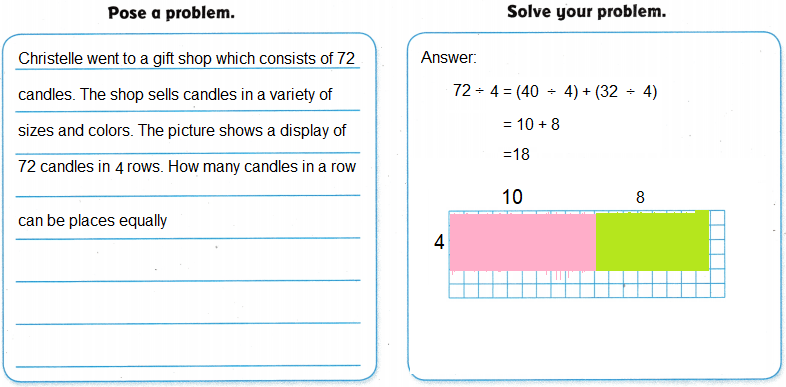Explanation:
Christelle went to gift shop consists of 72 candles.
The picture shows a display of 72 candles in 4 rows
Number of equal rows placed
72 ÷ 4 = 18

Question 6.
Apply During the day on Mercury, the temperature can reach 816°F, which is 6 times warmer than the highest temperature found on Earth. What temperature is the highest found on Earth?
(A) 129°F
(B) 132°F
(C) 136°F
(D) 137°F
Option (C)
Explanation:
During the day on Mercury, the temperature can reach 816°F,
which is 6 times warmer than the highest temperature found on Earth.
The highest temperature found on Earth
816 ÷ 6 =136

Question 7.
The chorus has 72 singers. The singers practice in 3 groups of equal size. Which expression is same as 72 ÷ 3?
(A) (42 ÷ 3) + (30 ÷ 3)
(B) (45 ÷ 3) + (25 ÷ 3)
(C) (40 ÷ 3) ÷ (30 ÷ 3)
(D) (57 ÷ 3) + (18 – 3)
Option(A)
Explanation:
The chorus has 72 singers.
The singers practice in 3 groups of equal size.
The possible way to break apart the model
72 ÷ 3 = (42 ÷ 3) + (30 ÷ 3)

Go Math Lesson 9.5 4th Grade Distributive Property of Division Question 8.
Multi-Step Analyze Terrance needs $150 to buy a bike. He has$36 saved. If he earns an equal amount over each of the next 3 weeks, how much must he earn each week to save enough for his bike?
(A) $50 (B)$40
(C) $38 (D)$62
Option(C)
Explanation:
Terrance needs $150 to buy a bike. He has$36 saved.
150 – 36 = 114
If he earns an equal amount over each of the next 3 weeks
114 ÷ 3 = $38 each week TEXAS Test Prep Question 9. Max had 200 baseball cards. He gave 14 of them to his younger brother. Max wants to arrange his remaining cards in equal rows of 6 cards each. How many rows of cards will Max have? (A) in 35 rows (B) in 31 rows (C) in 33 rows (D) in 36 rows Answer: Explanation: Max had 200 baseball cards. He gave 14 of them to his younger brother. 200 – 14 = 186 Max wants to arrange his remaining cards in equal rows of 6 cards each. 186 ÷ 6 = 31 rows. Texas Go Math Grade 4 Lesson 9.5 Homework and Practice Answer Key Model the division on the grid. Question 1. 48 ÷ 4 = (__________ ÷ 4) + (_________ ÷ 4) = _________ + _________ = _________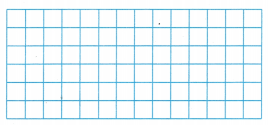Answer: 48 ÷ 4 = (40 ÷ 4) + (8 ÷ 4) = 10 + 2 = 12Explanation: Break apart the model into two rectangles to show 48 ÷ 4 = (40 ÷ 4) + (8 ÷ 4) = 10 + 2 = 12 Label and shade the smaller rectangles. Use two different colors to identify the groups. Question 2. 36 ÷ 3 = (__________ ÷ 3) + (__________ ÷ 3) = ___________ + ___________ = __________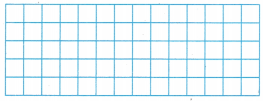Answer: 36 ÷ 3 = (30 ÷ 3) + (6 ÷ 3) = 10 + 2 = 12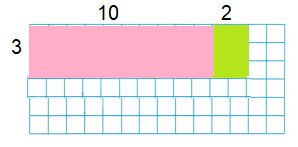Explanation: Break apart the model into two rectangles to show 36 ÷ 3 = (30 ÷ 3) + (6 ÷ 3) = 10 + 2 = 12 Label and shade the smaller rectangles. Use two different colors to identify the groups. Lesson 9.5 Answer Key Go Math 4th Grade Question 3. 28 ÷ 2 = (________ ÷ 2) + (________ ÷ 2) = __________ + _________ = ___________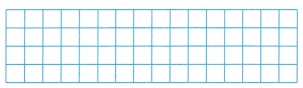Answer: 28 ÷ 2 = (20 ÷ 2) + (8 ÷ 2) = 10 + 4 = 14Explanation: Break apart the model into two rectangles to show 28 ÷ 2 = (20 ÷ 2) + (8 ÷ 2) = 10 + 4 = 14 Label and shade the smaller rectangles. Use two different colors to identify thee groups Question 4. 48 ÷ 3 = (_______ ÷ 3) + (_______ ÷ 3) = _________ + ________ = _________Answer: 48 ÷ 3 = (30 ÷ 3) + (18 ÷ 3) = 10 + 6 = 16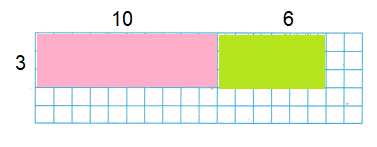Explanation: Break apart the model into two rectangles to show 48 ÷ 3 = (30 ÷ 3) + (18 ÷ 3) = 10 + 6 = 16 Label and shade the smaller rectangles. Use two different colors to identify thee groups. Problem Solving Question 5. There are 69 jobs for workers at the amusement park. There are 3 workers for each ride. Flow many rides are there? Answer: 23 riders. Explanation: There are 69 jobs for workers at the amusement park. There are 3 workers for each ride. Number of rides 69÷3 = 23 Question 6. The music club needs to sell 856 raffle tickets in order to buy new music stands. If each of the 8 members in the club wants to sell the same number of tickets, how many tickets does each member need to sell? Answer: 107 tickets. Explanation: The music club needs to sell 856 raffle tickets in order to buy new music stands. If each of the 8 members in the club wants to sell the same number of tickets, Number of tickets each member need to sell 856÷8=107 Lesson Check Fill in the bubble completely to show your answer. Question 7. Mr. Dominguez divided 68 students into 4 groups of equal size. Which of the following correctly uses the Distributive Property to find the number of students in each group? (A) (30 ÷ 4) + (28 ÷ 4) (B) (60 ÷ 4) + (10 ÷ 4) (C) (3o ÷ 4) + (3o ÷ 4) (D) (32 ÷ 4) + (36 ÷ 4) Answer: Option(D) Explanation: Mr. Dominguez divided 68 students into 4 groups of equal size. (32 ÷ 4) + (36 ÷ 4) 8 + 9 = 17 The number of students in each group is 17 Lesson 9.5 4th Grade Answers Go Math Question 8. The store clerk divided 168 shirts equally onto 4 different display tables. How many shirts did the clerk place on each table? (A) 32 (B) 44 (C) 42 (D) 31 Answer: Option(C) Explanation: The store clerk divided 168 shirts equally onto 4 different display tables. Number of shirts the clerk place on each table 168÷4 = 42 Question 9. On his vacation, Walter drove 780 miles. If he drove an equal number of miles each day for 6 days, how mans’ miles did he drive each day? (A) 130 miles (B) 140 miles (C) 120 miles (D) 150 miles Answer: Option(A) Explanation: Walter drove 780 miles. If he drove an equal number of miles each day for 6 days, Total miles he drive each day 780 ÷ 6 = 130 Question 10. A bait shop placed 128 worms into cups to sell. If there were 8 worms in each cup, how many cups of worms were there? (A) 24 (B) 16 (C) 12 (D) 18 Answer: Option(B) Explanation: A bait shop placed 128 worms into cups to sell. If there were 8 worms in each cup, Total cups of worms 128 ÷ 8 = 16 Question 11. Multi-Step Justin earned$50 mowing yards and $34 washing cars. He wants to divide his money into 3 equal accounts. How much will he put in each account? (A)$14
(B) $18 (C)$24
(D) $28 Answer: Option(D) Explanation: Justin earned$50 mowing yards and $34 washing cars. Total earned 50 + 34 =$84
He wants to divide his money into 3 equal accounts
Total amount he put in each account
84 ÷ 3 = $28 Go Math Practice and Homework Lesson 9.5 Answer Key 4th Grade Question 12. Multi-Step Kristen needs$325 to buy a plane ticket. She has saved $277. If she saves an equal amount each week over the next 4 weeks, how much must she save each week to have enough for the ticket? (A)$48
(B) $12 (C)$44
(D) $14 Answer: Option(B) Explanation: Kristen needs$325 to buy a plane ticket.
She has saved $277.$325 – $277 =$48
If she saves an equal amount each week over the next 4 weeks,
48÷4 = \$12

Scroll to Top